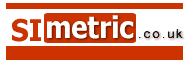Calculator for converting metres to feet, inches and tenths Conversion results from meters, millimetres or centimeters

 To convert metres to feet , inches and tenths of an inch or feet to metres Enter amount in metres (m) or, amount in centimetres (cm) or, amount in millimetres (mm) For result below in ft. ins. & fractions:-
 Some other associated conversions - 1 kilometre ( km ) = 1000 metre , 1 metre = 100 cm , 1 cm = 10 mm 1 mile = 5280 feet , 1 yard ( yd ) = 3 feet , 1 fathom = 6 feet , 1 chain = 66 feet 1 foot (ft.) = 12 inches (in.) To convert decimal fractions of an inch to fractions of an inch. Take the decimal fraction of feet and divide by 0.08333 (1/12th) and this will give you inches and decimals of an inch. For example - 6.37 feet. Take the 0.37 feet and divide by 0.0833 = 4.44 inches so for 6.37 ft. we can also say 6 ft.- 4.44 in. Now take the 0.44 in. and round it down to 0.4 which is 4/10" We can now say 6.37' approximatly equals 6' - 4 4/10" For fractions of an inch other than tenths, take the decimal remainder of inches and divide by:- 0.125 for the number of eighths 0.0625 for the number of sixteenths 0.03125 for the number of thirty-seconds 0.015625 for the number of sixty-fourths, and so on. For example - 4.382 inches. For eighths, divide the remainder, 0.382 by 0.125 and the answer is 3.056 so the fraction is a bit over 3 eighths, therefore the answer ends up as approx. 4 3/8" 1 millimetre is about one twenty-fifth of an inch ( 1 in. = 25.4 mm ) Feet may be shown as ft. or ' and inches as in. or "